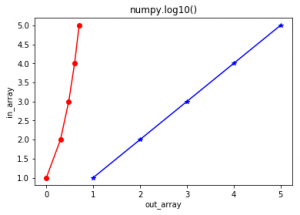# numpy.log10() in Python

numpy.log10(arr, out = None, *, where = True, casting = ‘same_kind’, order = ‘K’, dtype = None, ufunc ‘log10’) : This mathematical function helps user to calculate Base-10 logarithm of x where x belongs to all the input array elements.

Parameters :

```array    : [array_like]Input array or object.
out      : [ndarray, optional]Output array with same dimensions as Input array,
placed with result.
**kwargs : allows you to pass keyword variable length of argument to a function.
It is used when we want to handle named argument in a function.
where    : [array_like, optional]True value means to calculate the universal
functions(ufunc) at that position, False value means to leave the value in the
output alone.
```

Return :

```An array with Base-10 logarithmic value of x;
where x belongs to all elements of input array.
```

Code 1 : Working

 `# Python program explaining ` `# log10() function ` ` `  `import` `numpy as np ` ` `  `in_array ``=` `[``1``, ``3``, ``5``, ``10``*``*``8``] ` `print` `(``"Input array : "``, in_array) ` ` `  `out_array ``=` `np.log10(in_array) ` `print` `(``"Output array : "``, out_array) ` ` `  ` `  `print``(``"\nnp.log10(4**4) : "``, np.log10(``100``*``*``4``)) ` `print``(``"np.log10(2**8) : "``, np.log10(``10``*``*``8``)) `

Output :

```Input array :  [1, 3, 5, 100000000]
Output array :  [ 0.          0.47712125  0.69897     8.        ]

np.log10(4**4) :  8.0
np.log10(2**8) :  8.0
```

Code 2 : Graphical representation

 `# Python program showing ` `# Graphical representation of  ` `# log10() function ` ` `  `import` `numpy as np ` `import` `matplotlib.pyplot as plt ` ` `  `in_array ``=` `[``1``, ``2``, ``3``, ``4``, ``5``] ` `out_array ``=` `np.log10(in_array) ` ` `  `print` `(``"out_array : "``, out_array) ` ` `  `plt.plot(in_array, in_array, color ``=` `'blue'``, marker ``=` `"*"``) ` ` `  `# red for numpy.log10() ` `plt.plot(out_array, in_array, color ``=` `'red'``, marker ``=` `"o"``) ` `plt.title(``"numpy.log10()"``) ` `plt.xlabel(``"out_array"``) ` `plt.ylabel(``"in_array"``) ` `plt.show()   `

Output :

`out_array :  [ 0.          0.30103     0.47712125  0.60205999  0.69897   ]`References :
https://docs.scipy.org/doc/numpy-1.13.0/reference/generated/numpy.log10.html#numpy.log10
.

My Personal Notes arrow_drop_upIf you like GeeksforGeeks and would like to contribute, you can also write an article using contribute.geeksforgeeks.org or mail your article to contribute@geeksforgeeks.org. See your article appearing on the GeeksforGeeks main page and help other Geeks.

Please Improve this article if you find anything incorrect by clicking on the "Improve Article" button below.

Article Tags :

Be the First to upvote.

Please write to us at contribute@geeksforgeeks.org to report any issue with the above content.